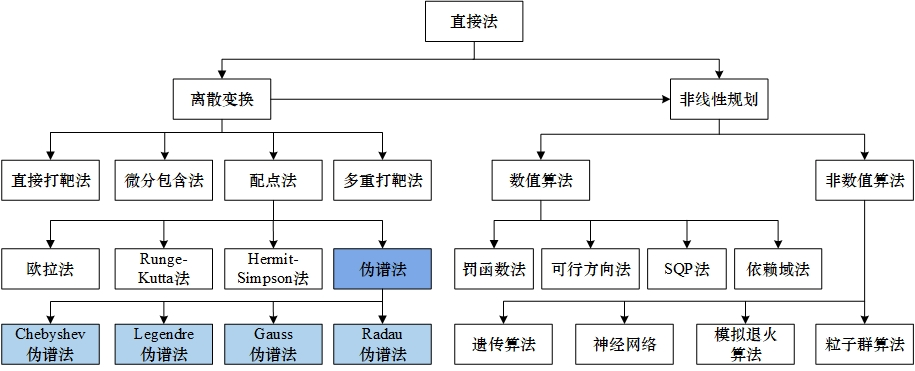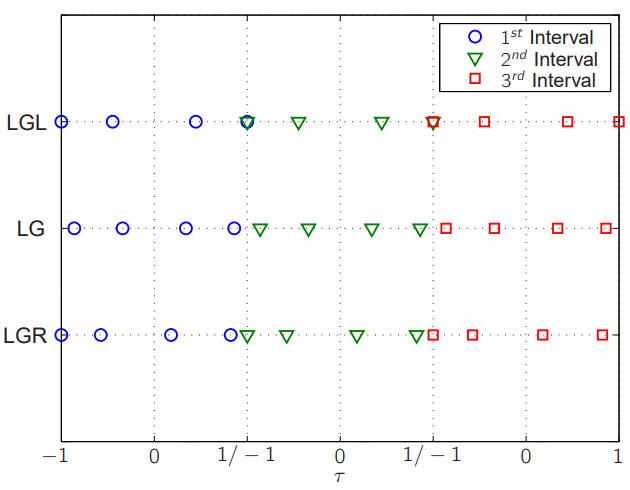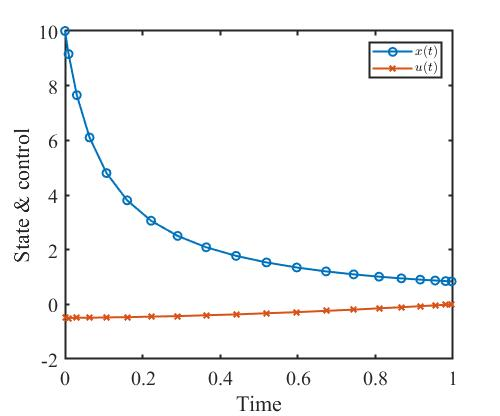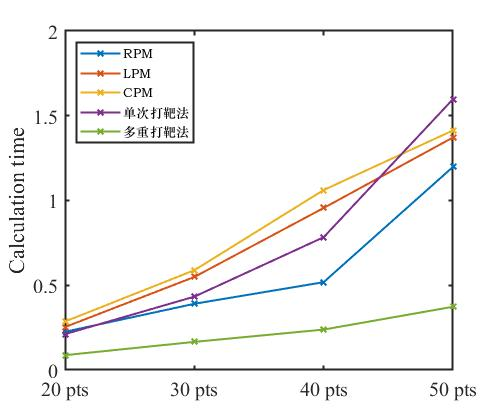# 写在前面

• 欧拉法；
• Runge-Kutta 法；
• Hermit-Simpson 法；
• 伪谱法（pseudospectral method）。# 伪谱法

• Chebyshev-Gauss-Lobatto（CGL）点；
• Legendre-Gauss-Lobatto（LGL）点；
• Legendre-Gauss（LG）点；

• 采用 CGL 点的称为 Chebyshev 伪谱法（CPM）。
• 采用 LGL 点的称为 Lobatto 伪谱法（LPM），也称为 Legendre 伪谱法；
• 采用 LG 点的称为 Gauss 伪谱法（GPM）；
• 采用 LGR 点的称为 Radau 伪谱法（RPM）。

## RPM 的原理

### 时域变换

\begin{aligned} \tau &= \frac{2t-t_f-t_0}{t_f-t_0}, \\ t &= \frac{t_f-t_0}{2}\tau + \frac{t_f+t_0}{2}. \end{aligned}

### 状态变量和控制变量的近似

RPM 选取$P_{n-1}(\tau) + P_n(\tau)$ 的零点，即 $n$ 阶 LGR 点 $\tau_n \ (n=1,2,\dots,N)$，以及 $\tau_0=-1$ 为节点，以 Lagrange 插值多项式为基函数近似状态变量和控制变量，为：

\begin{aligned} x(\tau) &\approx X(\tau) = \sum_{i=0}^N L_i(\tau)X(\tau_i), \\ u(\tau) &\approx U(\tau) = \sum_{k=1}^N \widetilde{L}_k(\tau) U(\tau_k), \end{aligned}

$P_n(x) = \sum_{m=0}^M (-1)^m \frac{(2n-2m)!}{2^n m! (n-m)! (n-2m)!} x^{n-2m},$

M = \left \{\begin{aligned} &\frac{n}{2}, &\quad 当\ n\ 为偶数时 \\ &\frac{n-1}{2}, &\quad 当\ n\ 为奇数时 \end{aligned} \right.

Legendre 多项式的 Rodrigues 表达式为：

$P_n(x) = \frac{1}{2^n n!} \frac{d^n}{x^n} (x^2-1)^n,$

Lagrange 插值多项式的数学描述为：

$L_i(\tau) = \prod_{j = 0,j \ne i}^N \frac{\tau - \tau_j}{\tau_i - \tau_j}, \quad i = 1,2,\dots,N,$

$L_i(\tau_j) = \left \{\begin{matrix} 1, \quad i = j, \\ 0, \quad i \ne j, \end{matrix} \right.$

Lagrange 插值多项式的插值误差为：

$E_N(\tau) = \frac{(\tau-\tau_1) \cdots (\tau-\tau_N)}{N!} L^N(\zeta),$

### 微分方程约束转化为代数约束

$\dot x(\tau_k) \approx \dot X(\tau_k) = \sum_{i=0}^N \dot{L}_i(\tau_k) X(\tau_i) = \sum_{i=0}^N D_{ki}X(\tau_i), \quad k = 1,2,\dots,K,$

D_{ki} = \dot L_i(\tau_k) = \left \{ \begin{aligned} &\frac{\dot g(\tau_k)}{(\tau_k-\tau_i)\dot g(\tau_i)}, & (k \ne i),\\ &\frac{\ddot g(\tau_k)}{2\dot g(\tau_i)}, & (k = i), \end{aligned} \right.

$g(\tau_i) = (1+\tau_i)[P_N(\tau_i)-P_{N-1}(\tau_i)], \quad i = 0,1,\dots,N,$

$F_k \equiv \sum_{i=0}^N D_{ki} X(\tau_i) - \frac{t_f-t_0}{2} f(X(\tau_i),U(\tau_i)) = 0, \quad k = 1,2,\dots,N.$

### 目标函数的近似

$J \approx \Phi(x)(\mathbf{x}(t_0),t_0,\mathbf{x}(t_f),t_f) + \frac{t_f-t_f}{2}\sum_{k=1}^N w_k g(X(\tau_k),U(\tau_k),\tau_k;t_0,t_f),$

$w_k = \int_{-1}^1 L_k(\tau) \text{d}t.$

### 约束条件的近似

$(F_1,F_2,\dots,F_N) = 0,$

$\phi(X(\tau_0),X(\tau_N)) = 0,$

$\mathbf{C}(X(\tau_k),U(\tau_k),\tau_k;t_0,t_f) \le 0, \quad k=1,2,\dots,N.$

## 各伪谱法特点

• 近似精度：GPM 和 RPM 在控制变量、状态变量和协态变量的近似精度优于 LPM。
• 收敛速度：LPM、GPM 和 RPM 的解均成指数规律收敛，但GPM 和 RPM 在收敛速度上优于LPM。
• 计算效率：对于相同规模问题的求解，LPM、GPM 和 RPM 耗时相差不大。
• 协态映射原理：GPM 和 RPM 是满足协态映射原理，LPM 不满足协态映射原理。
• 其他：与 GPM 相比，RPM 能够直接从相应的 NLP 问题的最优解中得到初始点的控制变量，而 GPM 不行，RPM 的这一性质使得在hp-伪谱法中采用 RPM 时，段与段的连续性方程可以略去，从而减少了约束方程的个数，使得 RPM 的实现比 GPM 简单。

## 各伪谱配点的分布特点• LG 点是 Legendre 多项式 $P_n(\tau)$ 的零点；
• LGR 点是 Legendre 多项式 $P_{n-1}(\tau) + P_n(\tau)$ 的零点；
• LGL 点是 Legendre 多项式 $P_{n-1}(\tau)$ 的驻点（导数为零的点）。

Legendre 多项式零点分布特点为中间稀疏、两边稠密。具体地，

• LG 点在 $[-1,1]$ 上不包含 $\tau=-1$ 和 $\tau=1$ 两个端点；
• LGR 点在 $[-1,1]$ 上包含一个端点，即包含 $\tau=-1$ 或 $\tau=1$；
• LGL 点在 $[-1,1]$ 上包含 $\tau=-1$ 和 $\tau=1$ 两个端点；## LGL 点不满足协态映射定理的原因

It is noted, however, that at the boundary points, the discrete equivalents of continuous boundary conditions (3–48) and (3–49) are coupled in the discrete costate dynamics in (3–98) and (3–99), respectively.

$\boldsymbol{\lambda}(-1) = -\nabla_{y(-1)}(\Phi-\langle \psi,\phi \rangle) \tag{3-48}$ $\boldsymbol{\lambda}(+1) = \nabla_{y(+1)}(\Phi-\langle \psi,\phi \rangle) \tag{3-49}$ \begin{aligned} \mathbf D_1^{\dagger } \boldsymbol{\widetilde{\lambda}} &=-\frac{t_f-t_0}{2} \nabla_{Y_1}(g_1+\langle \boldsymbol{\widetilde{\lambda}}_1, \mathbf{f}_1 \rangle-\langle \widetilde{\gamma}_1,\mathbf{C}_1 \rangle) \\ & - \frac{1}{w_1} \left ( \boldsymbol{\widetilde{\lambda}}_1 + \nabla_{Y_1}(\Phi-\langle \widetilde{\psi},\phi\rangle) \right ), \end{aligned} \tag{3-98} \begin{aligned} \mathbf D_N^{\dagger } \boldsymbol{\widetilde{\lambda}} &=-\frac{t_f-t_0}{2} \nabla_{Y_N}(g_N+\langle \boldsymbol{\widetilde{\lambda}}_N, \mathbf{f}_N \rangle-\langle \widetilde{\gamma}_N,\mathbf{C}_N \rangle) \\ & - \frac{1}{w_N} \left ( \boldsymbol{\widetilde{\lambda}}_N + \nabla_{Y_N}(\Phi-\langle \widetilde{\psi},\phi\rangle) \right ). \end{aligned} \tag{3-99}

LGL、LG、LGR 三种配点方式得到的配点见下图。# 算法步骤

1. 确定最优控制问题的离散点数、起止时间、子时间区间段数、状态变量初值、控制变量初值；
2. 根据不同配点方法求取配点；
3. 正确编写约束条件，这一步很重要，关系到伪谱法是否能够成功求解最优控制问题；
4. 将目标函数转化为离散形式；
5. 调用 matlab 的 fmincon() 求解该 NLP 问题。

# 代码

%--------------------------------------------------------------------------
% Method_Pseudospectral.m
% Attempt to solve the Bryson-Denham problem using a pseudospectral method
% (namely LGL nodes and Gaussian quadrature)
%--------------------------------------------------------------------------
%
%--------------------------------------------------------------------------
% Primary Contributor: Daniel R. Herber, Graduate Student, University of
% Illinois at Urbana-Champaign
% https://github.com/danielrherber/optimal-control-direct-method-examples
%--------------------------------------------------------------------------
%--------------------------------------------------------------------------
% 说明：应用伪谱法求解无约束最优控制问题
% 例子：《最优化与最优控制》 pp. 257 例13.1
% 类型：无控制约束的最优控制问题
% 时间：2022/07/07
%--------------------------------------------------------------------------
clear;clc;close all;

%% 01 初始参数设置
p.ns = 1; p.nu = 1;                     % 状态量个数和控制量个数
p.t0 = 0; p.tf = 1;                     % 初始时间和终止时间
p.x0 = 10;                              % 初始条件

p.nt = 20;                              % 配置点个数
% p.method = 'LGL';                       % 选择配点方式：Legendre-Gauss-Lobatto(LGL)
% p.method = 'CGL';                       % 或者 Chebyshev-Gauss-Lobatto(CGL)
p.method = 'LGR';                       % 或者 Legendre-Gauss-Radau(LGR)
if strcmp(p.method,'LGL')
p.tau = LGL_nodes(p.nt-1);          % 把时间区间离散到[-1,1]中
p.D   = LGL_Dmatrix(p.tau);         % 微分矩阵
p.w   = LGL_weights(p.tau);         % 配置点权重
elseif strcmp(p.method,'CGL')
p.tau = CGL_nodes(p.nt-1);          % 把时间区间离散到[-1,1]中
p.D   = CGL_Dmatrix(p.tau);         % 微分矩阵
p.w   = CGL_weights(p.tau);         % 配置点权重
elseif strcmp(p.method,'LGR')
[p.tau,p.w] = LGR_nodes_and_weights(p.nt-1);
p.D   = LGR_Dmatrix(p.tau);         % 微分矩阵
end

% 将变量离散为 x = [x,u]
p.x_index = 1:p.nt;
p.u_index = p.nt+1:2*p.nt;

%% 02 求解算法
% 给[x;u]赋初值
y0 = zeros(p.nt*(p.ns+p.nu),1);
% 求解器设置
options = optimoptions(@fmincon,'display','iter','MaxFunEvals',1e5,'algorithm','sqp');

tic;
[y,fval] = fmincon(@(y) objective(y,p),y0,[],[],[],[],[],[],@(y) constraints(y,p),options);
toc;

% 得到求解结果
p.x = y(p.x_index);                     % 状态量
p.u = y(p.u_index);                     % 控制量
p.t = tau2t(p);                         % 把时间变量从 tau 转化为 t

%% 03 画图
window_width = 500;
window_height = 416;

% 状态量
k = 1;
figure('color',[1 1 1],'position',[300+k*window_width,300,window_width,window_height]);
plot(p.t, p.x, 'o-', 'LineWidth',1.5);hold on;
plot(p.t, p.u, 'x-', 'LineWidth',1.5);
xlabel('Time');
ylabel('State & control');
set(gca,'FontSize',15,...
'FontName','Times New Roman',...
'LineWidth',1.5);
legend('$x(t)$','$u(t)$',...
'Location','Northeast',...
'FontSize',10,...
'interpreter','latex');

% 保存数据
% .\ 下一级文件夹
% ..\ 上一级文件夹
% save(['..\','pseudospectral_method.mat']);
%% 04 子函数部分
% 目标函数
function J = objective(y,p)
% 得到状态量和控制量
x = y(p.x_index);
u = y(p.u_index);

L = u.^2 + x.^2;                    % 积分项
J = (p.tf-p.t0)/2 * dot(p.w,L)/2;   % 计算目标函数
end

% 约束函数
function [c,ceq] = constraints(y,p)
% 得到状态量和控制量
x = y(p.x_index);
u = y(p.u_index);
% 状态方程约束
Y = x; F = (p.tf-p.t0)/2*(-x.^2+u);
% 初始状态约束
ceq1 = x(1) - p.x0;
ceq2 = p.D*Y - F;
% 输出约束条件
ceq = [ceq1;ceq2(:)];
c = [];
end

% 把 tau 转换为 t
function t = tau2t(p)
% 按照《天基对地打击武器轨道规划与制导技术》2013 pp.79 式(4.25)编写如下代码
t = (p.tf-p.t0)*p.tau/2+(p.tf-p.t0)/2;
end


# 结果# 对比

• 处理器：Intel(R) Core(TM) i5-8265U CPU @ 1.60GHz x86
• 内存： DD4R 2400 MHz 8GB + DD4R 2667 MHz 8GB
• 电源模式：平衡模式RPM LPM CPM 单次打靶法 多重打靶法
20 点 0.2255 0.2535 0.2861 0.2116 0.0875
30 点 0.3905 0.5488 0.5888 0.4328 0.1659
40 点 0.5183 0.9558 1.0617 0.7834 0.2385
50 点 1.1985 1.3719 1.4135 1.5981 0.3731

# 总结

1. Garg D. Advances in global pseudospectral methods for optimal control[D]. University of Florida, pp.75, 2011.